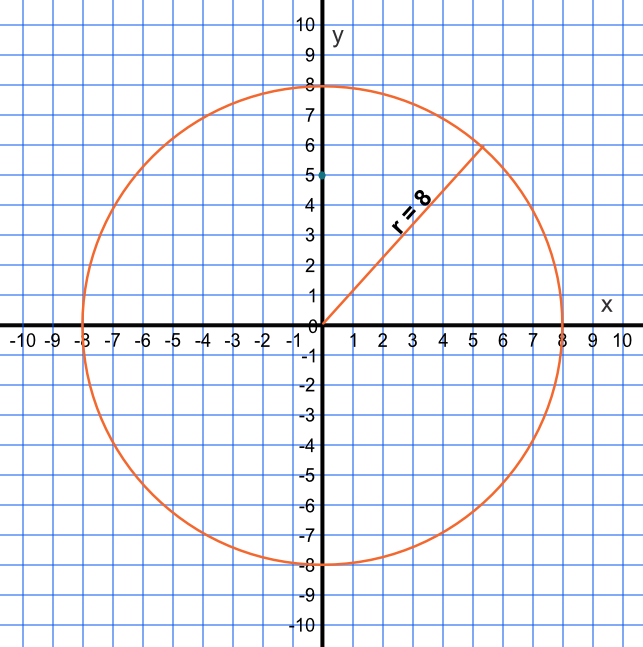Graph of a Circle

## Graph of a Circle

Pythagoras Theorem states that the sum of the square of the two shorter sides is equal to the square of the hypotenuse:

a^2 + b^2 = c^2

where a and b are the two shorter sides, and c is the long side.

Replace the a and b with x and y coordinates. Setting x^2 + y^2 = r^2, and keeping r constant, describes a circle of radius r with a centre at the origin.

## Example 1

A function is defined as x^2 + y^2 = 64. Construct the graph.

The radius of the circle is sqrt64. The centre of the circle is at 0,0, with radius 8.

Answer:## Example 2

A function is defined as x^2 + y^2 = 50. Is the coordinate (4, 3) within the circle?

Work out the value of 4^2 + 3^2 = 25. This is less than 50, so the coordinate is within the circle.